A piecewise linear function is a function composed of straight line segments. It is a piecewise function whose pieces are affine (linear) functions.

The graph of a piecewise linear functions consists of line segments and rays.

We have already study two basic examples: step functions and continuos piecewise linear functions.A piecewise function is a function that is defined by several subfunctions. If each piece is a constant function then the piecewise function is called Piecewise constant function or Step function.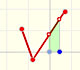A continuous piecewise linear function is defined by several segments or rays connected, without jumps between them.

A piecewise function could be piecewise continuous throughout its subdomains, but it could be not continuous on the entire domain. For example, a function can contains a jump discontinuity at some point.

Now we are going to study piecewise linear functions that do not need to be continuous. The graph of this kind of functions is made up of disconnected line segments. There are points on the graph of the function where a small change in x produces a sudden jump in the value of the function.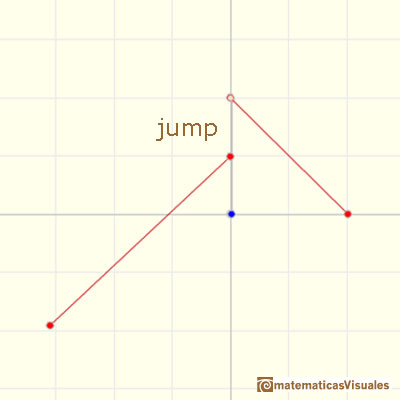We use discontinuos functions in lots of situations, for example, when we represent prices or taxes. It is not the same to buy one kilo of cafe than one thousand kilos: the price is not the same.

We remember that the graph of a linear function is an straight line.

The derivative of a linear function is a basic example of derivative, it is a constant function.The derivative of a lineal function is a constant function.

The derivative of this function (the segment slope) is a step function (notice that these functions are picewise differentiable, there is no derivative at the extrems of the subintervals).If we consider now the integral of this step function we get a continuous function. Each piece is a vertical translation of the original piece but the original function is not continuos at some points and the integral function is continuous. This is a consequence of the Fundamental Theorem of Calculus.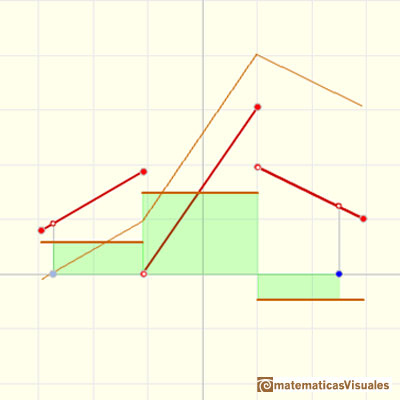If we change the lower limit of integration we get a vertical translation of the same integral function.Now we are going to study the integral of a piecewise linear function.

The integral of a non-constant linear function is a quadratic function.It is easy to calculate the area under a straight line. This is the first example of integration that allows us to understand the idea and to introduce several basic concepts: integral as area, limits of integration, positive and negative areas.You can play with a piecewise function f with two pieces:

The integral function F is made of several pieces that are quadratic (parabolas). It is a continuous function and piecewise differentiable.

But it is not smooth (it is not differentiable) where two pieces are connected.If the jump gets smaller then the integral function F becomes more and more smooth. We already know that if f is continuos then F is more than continuos, it is differentiable.Now you can play with a piecewise function with three pieces:

The average value of a function f(x) over the interval [a,b] is given by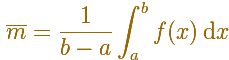In the next applet you can play with this concept when f(x) is a piecewise linear function.

As these functions are not, in general, continuous, you can not use the Mean Value Theorem for integrals and you CAN NOT say that there is a number c in [a,b] such thatLook at this example:REFERENCES

Tom M. Apostol, Calculus, Second Edition, John Willey and Sons, Inc.
Gilbert Strang, Sums and Differences vs. Integrals and Derivatives, The College Mathematics Journal, January 1990. JSTOR.
Anthony J. Macula, The Point-Slope Formula Leads to the Fundamental Theorem of Calculus, The College Mathematics Journal, Mathematical Association of America, 1995.
Michael Spivak, Calculus, Third Edition, Publish-or-Perish, Inc.As an introduction to Piecewise Linear Functions we study linear functions restricted to an open interval: their graphs are like segments.A piecewise function is a function that is defined by several subfunctions. If each piece is a constant function then the piecewise function is called Piecewise constant function or Step function.A continuous piecewise linear function is defined by several segments or rays connected, without jumps between them.Two points determine a stright line. As a function we call it a linear function. We can see the slope of a line and how we can get the equation of a line through two points. We study also the x-intercept and the y-intercept of a linear equation.Power with natural exponents are simple and important functions. Their inverse functions are power with rational exponents (a radical or a nth root)Polynomials of degree 2 are quadratic functions. Their graphs are parabolas. To find the x-intercepts we have to solve a quadratic equation. The vertex of a parabola is a maximum of minimum of the function.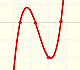Polynomials of degree 3 are cubic functions. A real cubic function always crosses the x-axis at least once.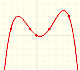We can consider the polynomial function that passes through a series of points of the plane. This is an interpolation problem that is solved here using the Lagrange interpolating polynomial.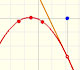The derivative of a quadratic function is a linear function, it is to say, a straight line.The derivative of a cubic function is a quadratic function, a parabola.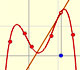Lagrange polynomials are polynomials that pases through n given points. We use Lagrange polynomials to explore a general polynomial function and its derivative.If the derivative of F(x) is f(x), then we say that an indefinite integral of f(x) with respect to x is F(x). We also say that F is an antiderivative or a primitive function of f.It is easy to calculate the area under a straight line. This is the first example of integration that allows us to understand the idea and to introduce several basic concepts: integral as area, limits of integration, positive and negative areas.To calculate the area under a parabola is more difficult than to calculate the area under a linear function. We show how to approximate this area using rectangles and that the integral function of a polynomial of degree 2 is a polynomial of degree 3.The integral of power functions was know by Cavalieri from n=1 to n=9. Fermat was able to solve this problem using geometric progressions.We can see some basic concepts about integration applied to a general polynomial function. Integral functions of polynomial functions are polynomial functions with one degree more than the original function.The integral concept is associate to the concept of area. We began considering the area limited by the graph of a function and the x-axis between two vertical lines.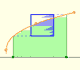Monotonic functions in a closed interval are integrable. In these cases we can bound the error we make when approximating the integral using rectangles.If we consider the lower limit of integration a as fixed and if we can calculate the integral for different values of the upper limit of integration b then we can define a new function: an indefinite integral of f.The Fundamental Theorem of Calculus tell us that every continuous function has an antiderivative and shows how to construct one using the integral.The Second Fundamental Theorem of Calculus is a powerful tool for evaluating definite integral (if we know an antiderivative of the function).Archimedes show us in 'The Method' how to use the lever law to discover the area of a parabolic segment.